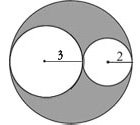Over Age 30 and Taking the GRE? Learn These 3 Key Things!   Get the FREE Video!

# Finding the Area of a Shaded Region on the GREA common GRE problem solving question asks the test-taker to find the area of a shaded region. For example, in the figure to the right, how would you find the area of the shaded region?

Unlike finding the area of a traditional geometric shape (e.g. triangle or trapezoid) for which you know a specific formula, there won’t be a specific formula you’ll need to learn to solve these types of questions. The shaded areas tend to be bizarre shapes, as you see in this figure, and thus a bit of creativity is required on your part.

The good news for you is that the way to go about solving these shaded region problems is always the same. Instead of trying to find the area of the shaded region directly, you’ll want to subtract the area of one of the shapes from the area of the other shape(s), and whatever is left over is the area of the shaded region. In other words:

Rule: To find the area of a shaded region, subtract the area of the smaller figure(s) (for which you have a known formula) from the area of the larger figure (for which you’ll also have a known formula).

In the figure above, for example,

The Area of the shaded region = (Area of the largest circle) – (Area of the circle with radius 3) – (Area of the circle with radius 2). Whatever is left over is the shaded region.

The diameter of the largest circle is 10, so its radius is 5 and thus its area is 25π.

Therefore, the Area of the shaded region = 25π – 9π – 4π = 12π

### On the GRE

To gain a deeper understanding of this concept and to see how it applies to a real GRE question, watch this video tutorial about finding the area of shaded regions on the GRE: